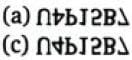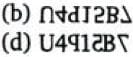Test Description

## 15 Questions MCQ Test Science Olympiad Class 9 | Olympiad Test: Water-Images

Olympiad Test: Water-Images for Class 9 2023 is part of Science Olympiad Class 9 preparation. The Olympiad Test: Water-Images questions and answers have been prepared according to the Class 9 exam syllabus.The Olympiad Test: Water-Images MCQs are made for Class 9 2023 Exam. Find important definitions, questions, notes, meanings, examples, exercises, MCQs and online tests for Olympiad Test: Water-Images below.
 1 Crore+ students have signed up on EduRev. Have you?
Olympiad Test: Water-Images - Question 1

### In each of the following question, a word is followed by four alternatives (a), (b), (c), (d) showing possible water images of that word. One out of these four alternatives show the exact water-image of that word. Choose the alternative which shows the correct water image of that word. CORDIAL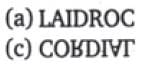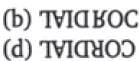Olympiad Test: Water-Images - Question 2

### In each of the following question, a word is followed by four alternatives (a), (b), (c), (d) showing possible water images of that word. One out of these four alternatives show the exact water-image of that word. Choose the alternative which shows the correct water image of that word. SUPERFLOUS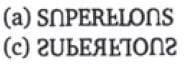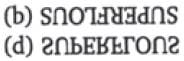Olympiad Test: Water-Images - Question 3

### In each of the following question, a word is followed by four alternatives (a), (b), (c), (d) showing possible water images of that word. One out of these four alternatives show the exact water-image of that word. Choose the alternative which shows the correct water image of that word. FECUND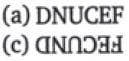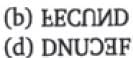Olympiad Test: Water-Images - Question 4

In each of the following question, a word is followed by four alternatives (a), (b), (c), (d) showing possible water images of that word. One out of these four alternatives show the exact water-image of that word. Choose the alternative which shows the correct water image of that word.

MUNDANE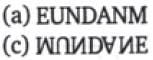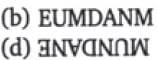Olympiad Test: Water-Images - Question 5

In each of the following question, a word is followed by four alternatives (a), (b), (c), (d) showing possible water images of that word. One out of these four alternatives show the exact water-image of that word. Choose the alternative which shows the correct water image of that word.

SARCASM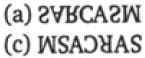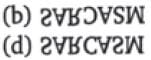Olympiad Test: Water-Images - Question 6

In each of the following question, a word is followed by four alternatives (a), (b), (c), (d) showing possible water images of that word. One out of these four alternatives show the exact water-image of that word. Choose the alternative which shows the correct water image of that word.

QUESTION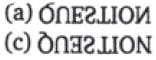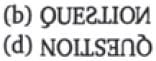Olympiad Test: Water-Images - Question 7

In each of the following question, a word is followed by four alternatives (a), (b), (c), (d) showing possible water images of that word. One out of these four alternatives show the exact water-image of that word. Choose the alternative which shows the correct water image of that word.

EXPOSE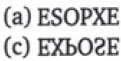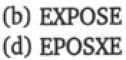Olympiad Test: Water-Images - Question 8

In each of the following question, a word is followed by four alternatives (a), (b), (c), (d) showing possible water images of that word. One out of these four alternatives show the exact water-image of that word. Choose the alternative which shows the correct water image of that word.

TERMINATE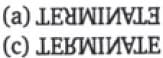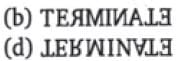Olympiad Test: Water-Images - Question 9

In each of the following question, a word is followed by four alternatives (a), (b), (c), (d) showing possible water images of that word. One out of these four alternatives show the exact water-image of that word. Choose the alternative which shows the correct water image of that word.

CLOSELY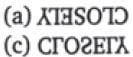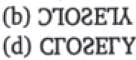Olympiad Test: Water-Images - Question 10

In each of the following question, a word is followed by four alternatives (a), (b), (c), (d) showing possible water images of that word. One out of these four alternatives show the exact water-image of that word. Choose the alternative which shows the correct water image of that word.

DARPAN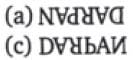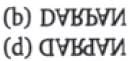Olympiad Test: Water-Images - Question 11

In each of the following question, a word is followed by four alternatives (a), (b), (c), (d) showing possible water images of that word. One out of these four alternatives show the exact water-image of that word. Choose the alternative which shows the correct water image of that word.

5DOB6V2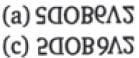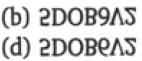Olympiad Test: Water-Images - Question 12

In each of the following question, a word is followed by four alternatives (a), (b), (c), (d) showing possible water images of that word. One out of these four alternatives show the exact water-image of that word. Choose the alternative which shows the correct water image of that word.

National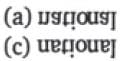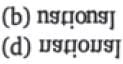Olympiad Test: Water-Images - Question 13

In each of the following question, a word is followed by four alternatives (a), (b), (c), (d) showing possible water images of that word. One out of these four alternatives show the exact water-image of that word. Choose the alternative which shows the correct water image of that word.

D6Z7F4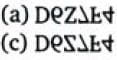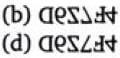Olympiad Test: Water-Images - Question 14

In each of the following question, a word is followed by four alternatives (a), (b), (c), (d) showing possible water images of that word. One out of these four alternatives show the exact water-image of that word. Choose the alternative which shows the correct water image of that word.

ACOUSTIC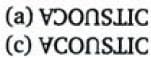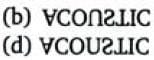Olympiad Test: Water-Images - Question 15

In each of the following question, a word is followed by four alternatives (a), (b), (c), (d) showing possible water images of that word. One out of these four alternatives show the exact water-image of that word. Choose the alternative which shows the correct water image of that word.

U4P15B7